# RD Sharma Solutions Class 7 Chapter 22 Data Handling Collection and Organisation of Data

Read RD Sharma Solutions Class 7 Chapter 22 Data Handling Collection and Organisation of Data below, students should study RD Sharma class 7 Mathematics available on Studiestoday.com with solved questions and answers. These chapter wise answers for class 7 Mathematics have been prepared by teacher of Grade 7. These RD Sharma class 7 Solutions have been designed as per the latest NCERT syllabus for class 7 and if practiced thoroughly can help you to score good marks in standard 7 Mathematics class tests and examinations

Exercise 22.1

Question :1. Define the following terms:

(i) Observations

(ii) Data

(iii) Frequency of an observation

(iv) Frequency distribution

Solution 1:

(i) Observations: The act of paying close attention to someone or something in order to obtain numerical data is known as observation.

(ii) Data: Data is a term that refers to a series of observations.

(iii) Frequency of an observation: The frequency of an observation is the number of times it occurs in a given set of data.

(iv) Frequency distribution: It's a way of viewing raw data in a way that's simple to comprehend.

Question :2. The final marks in Mathematics of 30 students are as follows:

53, 61, 48, 60, 78, 68, 55, 100, 67, 90

75, 88, 77, 37, 84, 58, 60, 48, 62, 56

44, 58, 52, 64, 98, 59, 70, 39, 50, 60

(i) Arrange these marks in the ascending order. 30 to 39 one group, 40 to 49 second group, etc.

(ii) What is the highest score?

(iii) What is the lowest score?

(iv) What is the range?

(v) If 40 is the pass mark how many have failed?

(vi) How many have scored 75 or more?

(vii) Which observations between 50 and 60 have not actually appealed?

(viii) How many have scored less than 50?

Solution 2:

(i) Groups the number in ascending order:

Group one             30 – 39 -      37, 39
Group two             40 – 49 -      44, 48, 48
Group three           50 – 59 -     50, 52, 53, 55, 56, 58, 58, 59
Group four             60 – 69 -     60, 60, 60, 61, 62, 64, 67, 68
Group five             70 – 79 -     70, 75, 77, 78
Group six              80 – 89 -     84, 88
Group seven         90 – 99 -      90, 98
Group eight          100 – 109 -  100

(ii) 100 is the highest score.

(iii) 37 is the lowest score.

(iv) Range is = Maximum observation – Minimum observation.

= 100 – 37

= 63.

(v) Just two students have failed if the pass mark is 40.

(vi) 8 students received a score of 75 or higher.

(vii) Between 50 and 60, there are no numbers 51, 54, or 57.

(viii) 5 students received a score of less than 50.

Question :3. The weights of new born babies (in kg) in a hospital on a particular day are as follows:

2.3, 2.2, 2.1, 2.7, 2.6, 3.0, 2.5, 2.9, 2.8, 3.1, 2.5, 2.8, 2.7, 2.9, 2.4

(i) Rearrange the weights in descending order.

(ii) Determine the highest weight.

(iii) Determine the lowest weight.

(iv) Determine the range.

(v) How many babies were born on that day?

(vi) How many babies weigh below 2.5 kg?

(vii) How many babies weigh more than 2.8?

(viii) How many babies weigh 2.8 kg?

Solution 3:

(i) Descending order of weights:

3.1, 3.0, 2.9, 2.9, 2.8, 2.8, 2.7, 2.7, 2.6, 2.5, 2.5, 2.4, 2.3, 2.2, 2.1

(ii) Highest weight is 3.1 Kg.

(iii) Lowest weight is 2.1 Kg.

(iv) Range = Maximum observation – Minimum observation

= (3.1-2.1) kg

= 1.0 Kg.

(v) On that particular day, 15 babies were born.

(vi) 4 of the babies are under 2.5 kg.

(vii) There are 4 babies weighing more than 2.8 kg.

(viii) There are 2 babies, each weighing 2.8 kg.

Question :4. Following data gives the number of children in 40 families:

1, 2, 6, 5, 1, 5, 1, 3, 2, 6, 2, 3, 4, 2, 0, 0, 4, 4, 3, 2

2, 0, 0, 1, 2, 2, 4, 3, 2, 1, 0, 5, 1, 2, 4, 3, 4, 1, 6, 2

Represent it in the form of a frequency distribution.

Solution 4:

Required frequency table for given data is:

Number of Children      Frequency
0                                     5
1                                     7
2                                    11
3                                     5
4                                     6
5                                     3
6                                     3

Question :5. Prepare a frequency table of the following scores obtained by 50 students in a test:

42        51       21       42       37       37       42       49       38       52

7          33       17       44       39      7         14        27        39        42

42        62       37       39       67       51       53        53        59        41

29        38       27       31       54        19       53        51        22        61

42        39       59       47       33        34       16        37        57        43

Solution 5:

Frequency-distribution table for given data: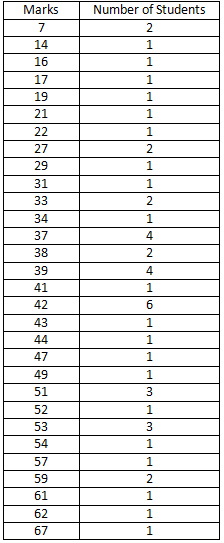Question :6. A die was thrown 25 times and following scores were obtained:

1          5          2          4          3

6          1          4          2          5

1          6          2          6          3

5          4          1          3          2

3          6          1          5          2

Prepare a frequency table of the scores.

Solution 6:

Frequency table: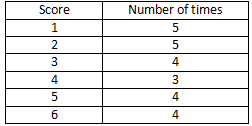Question :7. In a study of number of accidents per day, the observations for 30 days were obtained as follows:

6          3          5          6          4          3          2          5          4          2

4          2          1          2          2          0          5          4          6          1

6          0          5          3          6          1          5          5          2          6

Prepare a frequency distribution table.

Solution 7:

Frequency table for given data: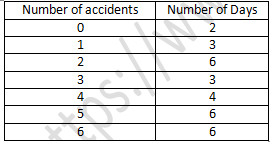Question :8. Prepare a frequency table of the following ages (in years) of 30 students of class VIII in your school:

13, 14, 13, 12, 14, 13, 14, 15, 13, 14, 13, 14, 16, 12, 14

13, 14, 15, 16, 13, 14, 13, 12, 17, 13, 12, 13, 13, 13, 14

Solution 8:

Frequency distribution table: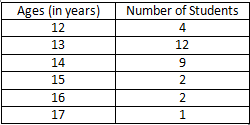Question :9. Following figures relate the weekly wages (in Rs) of 15 workers in a factory:

300, 250, 200, 250, 200, 150, 350, 200, 250, 200, 150, 300, 150, 200, 250

Prepare a frequency table.

(i) What is the range in wages (in Rs)?

(ii) How many Workers are getting Rs 350?

(iii) How many workers are getting the minimum wages?

Solution 9:

Frequency distribution table is:(i) The range in wages = Rs. 350 – Rs. 150 = Rs. 200.

(ii) Only 1 worker is getting Rs. 350.

(iii) 3 employees are paid the minimum wage, which is Rs. 150.

Question :10. Construct a frequency distribution table for the following marks obtained by 25 students in a history test in class VI of a school:

9, 17, 12, 20, 9, 18, 25, 17, 19, 9, 12, 9,12, 18, 17,19, 20,25, 9, 12, 17, 19, 19, 20, 9

(i) What is the range of marks?

(ii) What is the highest mark?

(iii) Which mark is occurring more frequently?

Solution 10:

Required frequency distribution table is:(i) Range of marks: 25 – 9 = 16.

(ii) 25 is the highest mark.

(iii) 9 is occurring more frequently.

Question :11. In a Mathematics test following marks were obtained by 40 students of class VI. Arrange these marks in a table using, tally marks.

8          1          3          7          6          5          5          4          4          2

4          9          5          3          7          1          6          5          2          7

7          3          8          4          2          6          9          5          8          6

7          4          5          6          9          6          4          4          6          6

(i) Find how many students obtained marks equal to or more than 7?

(ii) How many students obtained marks below 4?

Solution 11: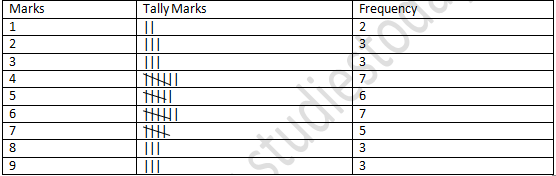(i) Arrange the names of sweets in a table using tally marks.

(ii) Which sweet is preferred by most of the students?

Solution 12: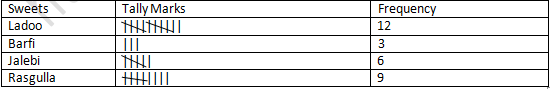(ii) The majority of the 12 students prefer ladoo.